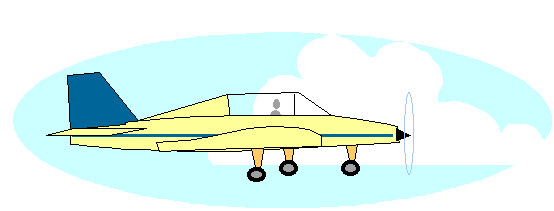+ Text Only Site
+ Non-Flash Version
+ Contact GlennVelocity and Distance for Constant Force Problems If so instructed by your teacher, print out a worksheet page for these problems. Click Beginner's Guide Index to access the list of slides. Open the slide called Simple Aircraft Motion (with text) and read the explanation of the equations of motion resulting from constant force and motion.Graph the equation v = at + v0 over the domain of 0 < t < 4 hours where a= 10 mph2 and v0= 100 mph. What does the velocity intercept represent?   Calculate the slope of the graph.   What does the slope represent?   What is your velocity after four hours? Does this make sense?   What happens to your graph if v0 = 0?   What effect does a change in acceleration a have upon your graph?   Using the equation x = 1/2 at2 + v0t + x0 with an x0 =50 miles, graph x over the domain of 0 < t < 4 hours. Is this a reasonable domain for this equation?

Related Pages:
Standards
Worksheet
Lesson Index
Aerodynamics Index+ Inspector General Hotline + Equal Employment Opportunity Data Posted Pursuant to the No Fear Act + Budgets, Strategic Plans and Accountability Reports + Freedom of Information Act + The President's Management Agenda + NASA Privacy Statement, Disclaimer, and Accessibility CertificationEditor: Tom Benson NASA Official: Tom Benson Last Updated: Fri, May 07 02:42:48 PM EDT 2021 + Contact Glenn